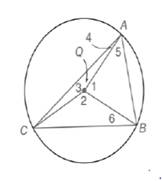Chapter 6.1, Problem 11E### Elementary Geometry for College St...

6th Edition
Daniel C. Alexander + 1 other
ISBN: 9781285195698

#### Solutions

Chapter
Section### Elementary Geometry for College St...

6th Edition
Daniel C. Alexander + 1 other
ISBN: 9781285195698
Textbook Problem
51 views

# Given: ⊙ Q  in which m A B ⌢ : m B C ⌢ : m C A ⌢ = 2 : 3 : 4Find: a) m A B ⌢ b) m B C ⌢ c) m C A ⌢ d) m ∠ 1( ∠ A Q B ) e) m ∠ 2 ( ∠ C Q B ) f) m ∠ 3 ( ∠ C Q A ) g) m ∠ 4 ( ∠ C A Q ) h) m ∠ 5 ( ∠ Q A B ) i) m ∠ 6 ( ∠ Q B C ) (HINT): Let m A B ⌢ = 2 x , m B C ⌢ = 3 x , and m C A ⌢ = 4 x . )

To determine

a)

To calculate:

The mAB by using the provided figure.

Explanation

Given:

The provided figure is given below where Q in which mAB:mBC:mCA=2:3:4.

Definition:

Since, the sum of the measures of the consecutive arcs that form a circle is 360o.

Calculation:

Let, the ratio in the form given below:

mAB=2xmBC=3xmCA=4x

Since, the sum of the measures of the consecutive arcs that form a circle is 360o.

To determine

b)

To calculate:

The mBC by using the provided figure.

To determine

c)

To calculate:

The mCA by using the provided figure.

To determine

d)

To calculate:

The m1(AQB) by using the provided figure.

To determine

e)

To calculate:

The m2(CQB) by using the provided figure.

To determine

f)

To calculate:

The m3(CQA) by using the provided figure.

To determine

g)

To calculate:

The m4(CAQ) by using the provided figure.

To determine

h)

To calculate:

The m5(QAB) by using the provided figure.

To determine

i)

To calculate:

The m6(QBC) by using the provided figure.

### Still sussing out bartleby?

Check out a sample textbook solution.

See a sample solution

#### The Solution to Your Study Problems

Bartleby provides explanations to thousands of textbook problems written by our experts, many with advanced degrees!

Get Started

#### The difference between population variance and sample variance.

Statistics for The Behavioral Sciences (MindTap Course List)

#### In Problems 19-26, find the derivative of each function. 25.

Mathematical Applications for the Management, Life, and Social Sciences

#### The solution to y = y2 with y(1)=13 is: a) y=1x+2 b) y=lnx+13 c) y=12x216 d) y=12x16

Study Guide for Stewart's Single Variable Calculus: Early Transcendentals, 8th